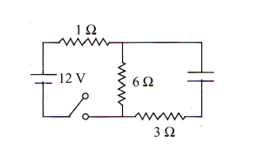Ohm's law
Question

# When the switch is closed, then initial current through 1 $\mathrm{\Omega }$ resistor is (see figure)Easy
Solution

## Initially cement through the capacitor will be  maximumSo 6 $\mathrm{\Omega }$ and 3 $\mathrm{\Omega }$ are in parallel total  resistance$\begin{array}{l}\frac{6×3}{9}+1=3\mathrm{\Omega }\\ \mathrm{i}=\frac{12}{3}=4\mathrm{\Omega }\end{array}$

Get Instant Solutions